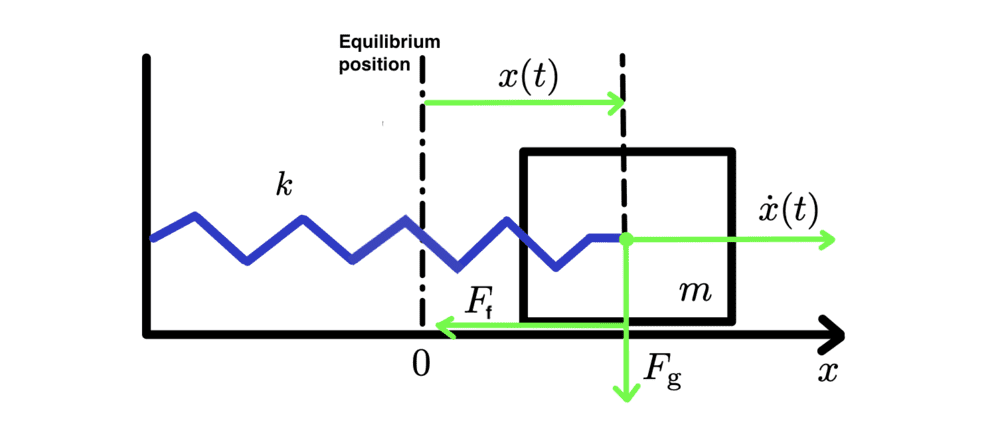# How to solve a differential equation for a mass-spring oscillator?

bolzano95
Homework Statement:
There is an mass-spring oscillator made of a spring with stiffness k and a block of mass m. The block is affected by a friction.
At the time ##t=0s## the block is pulled from the equilibrium position for ##x=5cm## in the right direction and released.
Derive and solve the equation for a displacement x, velocity v and power P.
Relevant Equations:
II. Newton's Law
There is an mass-spring oscillator made of a spring with stiffness k and a block of mass m. The block is affected by a friction given by the equation:
$$F_f = -k_f N tanh(\frac{v}{v_c})$$
##k_f## - friction coefficient
N - normal force
##v_c## - velocity tolerance.

At the time ##t=0s## the block is pulled from the equilibrium position for ##x=5cm## in the right direction and released.
1. Derive the differential equation.
2. Solve it for a displacement x.
3. Solve it for a velocity v.
4. Solve it for the power loss P, because of the friction.1. Deriving the necessary equation:
In the vertical direction: N=mg.
Because the only forces acting on the block in horizontal direction are the spring force and friction we can write:
$$-kx + k_f\, N\, tanh(\frac{\dot{x}}{v_c})=m\ddot{x}$$
$$m\ddot{x} - k_f\: mg\: tanh(\frac{\dot{x}}{v_c}) +kx=0$$

Here my solving stops, because I'm not sure how to implement the standard solution ##x(t)= Ce^{\lambda t}## because velocity is inside the function ##tanh(\frac{\dot{x}}{v_c})##.

Homework Helper
Gold Member
You are given a numerical value for the initial displacement. Are you given numerical values for any of the other parameters such as ##k, k_f, v_c## and ##m##?

At first glance, the differential equation looks difficult to solve unless you can make a small amplitude approximation such that ##\dot x## is always much less than ##v_c##.

bolzano95
You are given a numerical value for the initial displacement. Are you given numerical values for any of the other parameters such as ##k, k_f, v_c## and ##m##?

At first glance, the differential equation looks difficult to solve unless you can make a small amplitude approximation such that ##\dot x## is always much less than ##v_c##.
Oh yes, the initial conditions and parameters of the system given.

So there is no chance of solving this analytically, only numerically?